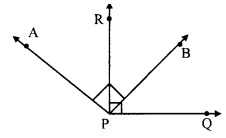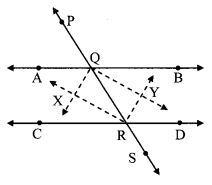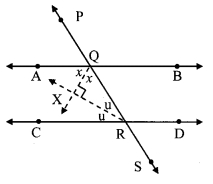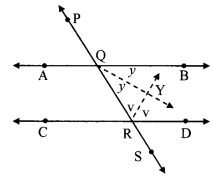# Maharashtra Board 9th Class Maths Part 2 Problem Set 2 Solutions Chapter 2 Parallel Lines

Balbharti Maharashtra State Board Class 9 Maths Solutions covers the Problem Set 2 Geometry 9th Class Maths Part 2 Answers Solutions Chapter 2 Parallel Lines.

## Problem Set 2 Geometry 9th Std Maths Part 2 Answers Chapter 2 Parallel Lines

Question 1.
Select the correct alternative and fill in the blanks in the following statements.

i. If a transversal intersects two parallel lines then the sum of interior angles on the same side of the transversal is ____.
(A) 0°
(B) 90°
(C) 180°
(D) 360°
(C) 180°

ii. The number of angles formed by a transversal of two lines is _____.
(A) 2
(B) 4
(C) 8
(D) 16
(C) 8

iii. A transversal intersects two parallel lines. If the measure of one of the angles is 40°, then the measure of its corresponding angle is ______.
(A) 40°
(B) 140°
(C) 50°
(D) 180°
(A) 40°

iv. In ∆ABC, ∠A = 76°, ∠B = 48°, then ∠C = _____.
(A) 66°
(B) 56°
(C) 124°
(D) 28°
In ∆ABC, ∠A + ∠B + ∠C = 180°
∴ ∠C = 180° – 76° – 48° = 56°
(B) 56°

v. Two parallel lines are intersected by a transversal. If measure of one of the alternate interior angles is 75° then the measure of the other angle is _____.
(A) 105°
(B) 15°
(C) 75°
(D) 45°
(C) 75°

Question 2.
Ray PQ and ray PR are perpendicular to each other. Points B and A are in the interior and exterior of ∠QPR respectively. Ray PB and ray PA are perpendicular to each other.
Draw a figure showing all these rays and write –
i. A pair of complementary angles
ii. A pair of supplementary angles
iii. A pair of congruent angles.
Solution:i. Complementary angles:
∠RPQ = 90° [Ray PQ ⊥ ray PR]
∴ ∠RPB + ∠BPQ = 90° [Angle addition property]
∠RPB and ∠BPQ are pair of complementary angles
∠APB = 90° [Ray PA ⊥ ray PB]
∴ ∠APR + ∠RPB = 90°
∠APR and ∠RPB are pair of complementary angles.

ii. Supplementary angles:
∠APB + ∠RPQ = 90° + 90° = 180°
∴ ∠APB and ∠RPQ are a pair of supplementary angles.

iii. Congruent angles:
a. ∠APB = ∠RPQ [Each is of 90°]
b. ∠APB = ∠RPQ
∴ ∠APR + ∠RPB = ∠RPB + ∠BPQ [Angle addition property]
∴ ∠APR = ∠BPQ
∴ ∠APR ≅ ∠BPQ

Question 3.
Prove that, if a line is perpendicular to one of the two parallel lines, then it is perpendicular to the other line also.Given: line AB || line CD and line EF intersects them at P and Q respectively.
line EF ⊥ line AB
To prove: line EF ⊥ line CD
Solution:
Proof:
line EF ⊥ line AB [Given]
∴ ∠APR = 90° ….(i)
line AB || line CD and line EF is their transversal.
∴ ∠EPB ≅ ∠PQD …..(ii) [Corresponding angles]
∴ ∠PQD = 90° [From (i) and (ii)]
∴ line EF ⊥ line CD

Question 4.
In the given figure, measures of some angles are shown. Using the measures find the measures of ∠x and ∠y and hence show that line l || line m.Solution:
Proof:
∠x = 130°
∠y = 50° [Vertically opposite angles]
Here, m∠PQT + m∠QTS = 130° + 50° = 180°
But, ∠ PQT and ∠ QTS are a pair of interior angles on lines l and m when line n is the transversal,∴ line l || line m [Interior angles test]

Question 5.
In the given figure, Line AB || line CD || line EF and line QP is their transversal. If y : z = 3 : 7 then find the measure of ∠x.Solution:
y : z = 3 : 7 [Given]
Let the common multiple be m
∴ ∠j = 3m and ∠z = 7m ….(i)
line AB || line EF and line PQ is their transversal [Given]
∠x = ∠z
∴ ∠x = 7m …..(ii) [From (i)]
line AB || line CD and line PQ is their transversal [Given]
∠x + ∠y = 180°
∴ 7m + 3m = 180°
∴ 10m = 180°
∴ m = 18°
∴ ∠x = 7m = 7(18°) [From (ii)]
∴ ∠x = 126°

Question 6.
In the given figure, if line q || line r, line p is their transversal and if a = 80°, find the values of f and g.Solution:
i. ∠a = 80° [Given]
∠g = ∠a [Alternate exterior angles]
∴ ∠g = 80° …..(i)

ii. Now, line q || line r and line p is their transversal.
∴ ∠f + ∠g = 180°
∴ ∠f + 80° = 180° [Interior angles]
∴ ∠f = 180° – 80° [From (i)]
∴ ∠f=100°

Question 7.
In the given figure, if line AB || line CF and line BC || line ED then prove that ∠ABC = ∠FDE.Given: line AB || line CF and line BC || line ED
To prove: ∠ABC = ∠FDE
Solution:
Proof:
line AB || line PF and line BC is their transversal.
∴ ∠ABC = ∠BCD ….(i) [Alternate angles]
line BC || line ED and line CD is their transversal.
∴ ∠BCD = ∠FDE ….(ii) [Corresponding angles]
∴ ∠ABC = ∠FDE [From (i) and (ii)]

Question 8.
In the given figure, line PS is a transversal of parallel line AB and line CD. If Ray QX, ray QY, ray RX, ray RY are angle bisectors, then prove that □ QXRY is a rectangle.Given: line AB || line CD
Rays QX, RX, QY, RY are the bisectors of ∠AQR, ∠QRC, ∠BQR and ∠QRD respectively.
To prove: □QXRY is a rectangle.
Proof:
∠XQA = ∠XQR = x° ……(i) [Ray QX bisects ∠AQR]
∠YQR = ∠YQB =y° …….(ii) [Ray QY bisects ∠BQR]
∠XRQ = ∠XRC = u° …….. (iii) [Ray RX bisects ∠CRQ]
∠YRQ = ∠YRD = v° ……(iv) [Ray RY bisects ∠DRQ]
line AB || line CD and line PS is their transversal.∠AQR+ ∠CRQ= 180° [Interior angles]
(∠XQA + ∠XQR) + (∠XRQ + ∠XRC) = 180° [Angle addition property]
∴ (x + x) + (u + u) = 180° [From (i) and (ii)]
∴ 2x + 2u = 180°
∴ 2(x + u) = 180°
∴ x + u = 90° ……..(v)
In ∆XQR
∠XQR + ∠XRQ + ∠QXR = 180° [Sum of the measures of the angles of triangle is 180°]
∴ x + u + ∠QXR = 180° [From (i) and (iii)]
∴ 90 + ∠QXR = 180° [From (v)]
∴ ∠QXR = 180°- 90°
∴ ∠QXR = 90° …..(vi)
Similarily we can prove that,∴ y + v = 90°
Hence ∠QYR = 90° ……(vii)
Now, ∠AQR + ∠BQR = 180° [Angles is linear pair]
(∠XQA + ∠XQR) + (∠YQR + ∠YQB) = 180° [Angle addition property]
∴ (x + x) + (y + y) = 180° [From (i) and (ii)]
∴ 2x + 2y = 180°
∴ 2(x+y) = 180°
∴ x +y = 90°
i.e. ∠XQR + ∠YQR = 90° [From (i) and (ii)]
∴ ∠XQY = 90° ….(viii) [Angle addition property]
Similarly we can prove that,
∠XRY = 90° …(ix)
In □QXRY
∠QXR = ∠QYR = ∠XQY = ∠XRY = 90° [From (vi), (vii), (viii) and (ix)]
∴□ QXRY is a rectangle.

Maharashtra Board Class 9 Maths Chapter 2 Parallel Lines Problem Set 2 Intext Questions and Activities

Question 1.
To verify the properties of angles formed by a transversal of two parallel lines. (Textbook pg. no. 14)
Take a piece of thick coloured paper. Draw a pair of parallel lines and a transversal on it. Paste straight sticks on the lines. Eight angles will be formed. Cut pieces of coloured paper, as shown in the figure, which will just fit at the comers of ∠1 and ∠2. Place the pieces near different pairs of corresponding angles, alternate angles and interior angles and verify their properties.